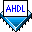## Using If Then Statement Logic

 See AlsoThe priority.tdf file shown below shows a priority encoder that converts the level of the highest-priority active input into a value. It generates a 2-bit code that indicates the highest-priority input driven by `VCC`.

```SUBDESIGN priority
(
low, middle, high   : INPUT;
highest_level[1..0] : OUTPUT;
)
BEGIN
IF high THEN
highest_level[] = 3;
ELSIF middle THEN
highest_level[] = 2;
ELSIF low THEN
highest_level[] = 1;
ELSE
highest_level[] = 0;
END IF;
END;
```

In this example, the inputs `high`, `middle`, and `low` are evaluated to determine whether they are driven by `VCC`. The If Then Statement activates the equations that follow the active `IF` or `ELSE` clause, that is, if `high` is driven by `VCC`, `highest_level[]` is `3`.

If more than one input is driven by `VCC`, the If Then Statement evaluates the priority of the inputs, which is determined by the order of the `IF` and `ELSIF` clauses (the first clause has the highest priority). In priority.tdf, `high `has the highest priority, `middle` has the next highest priority, and `low` has the lowest priority. The If Then Statement activates the equations that follow the highest-priority `IF` or `ELSE` clause that is true.

If none of the inputs are driven by `VCC`, the equations following the `ELSE` keyword are activated.